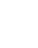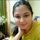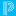## rounding of average up to two decimal placesJourneyman

## rounding of average up to two decimal places

How to round off these averages up to 2 decimal places in an object report - my dats and formula is

^(f.div;^(f.add;^(*period_info;P2(A) P4(B,H) P6(E,I) P8(J);current_percent);^(*period_info;P2(D,F,I) P3(B) P4(A) P6(J);current_percent);^(*period_info;P4(F) P5(H) P7(A,E,G) P8(B);current_percent);^(*period_info;P2(J) P4(G) P5(E) P6(C) P7(I) P8(A,D,F);current_percent);^(*period_info;P2(D) P5(A,E) P6(H,I) P8(J);current_percent);^(*period_info;P2(F) P3(B) P4(I) P6(J) P7(E) P8(G);current_percent);^(*period_info;P3(F,J) P4(E) P5(C) P7(B) P8(D);current_percent);^(*period_info;P2(B) P3(F) P4(E) P6(A,H) P7(D);current_percent);^(*period_info;P3(G) P5(B,D) P6(F) P7(J) P8(I);current_percent);^(*period_info;P2(E) P3(D,H) P4(C) P5(F,G);current_percent);^(*period_info;P4(D,J) P5(A,C,I) P8(G);current_percent););8)Community Support Expert

Hi @pankaj

We recommend reaching out to the Technical Contact of your district who should be able to connect with the Keys-to-Ownership(KTO) team. The Team further would be able to assist you with the object report and your other requirements.

KritiS
Community Moderator

Remember to give Kudos to suggestions that help you!
If a suggestion helps solve your issue, please select Accept As Solution on the post so others can see the solution, too!